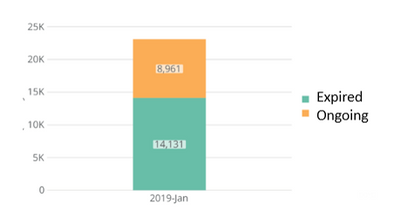# how to use the percentage of a category as the summary number

Hello, I have this card that looks like this (Showing only one month's data)What I want to show in the summary number is the percentage of expired category(14,131 / 8,961+14,131) that is made available when you hover over the chart (61.19%). How do I do that for the summary number? Thanks!

• You would need to create a Beast Mode basically says:

`SUM(CASE WHEN `Category` like 'Expired' THEN `Value` END)/SUM(`Value`)`

Then select that Beast Mode as your summary number. (one tip on summary numbers, they will only allow you to use a beast mode if the beast mode is an aggregation. Can't put something like 1+1 would instead need SUM(1+1))

Best of luck,
Valiant

**Say "Thanks" by clicking the "heart" in the post that helped you.

•CoachYou mentioned that your sample data was only showing one month.  Does that mean that your actual card typically shows multiple months?

In which case, the beast mode that @Valiant suggested will be calculating the % expired over the entire time period that you have selected.  If you only want to show one month's expired percentage then you could add some more conditions to the case statement.  If you want the summary number to focus on the current month:

`SUM(CASE WHEN `Category` like 'Expired' AND MONTH(`Date Field`)=MONTH(CURDATE()) THEN `Value` END)/SUM(CASE WHEN MONTH(`Date Field`)=MONTH(CURDATE()) THEN `Value` END)`

______________________________________________________________________________________________
“There is a superhero in all of us, we just need the courage to put on the cape.” -Superman
______________________________________________________________________________________________